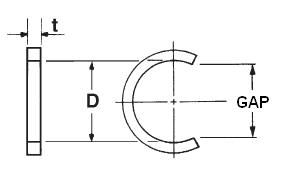### XSO-227

Display Units: inches |  metric
Request Quick Quote for this part.
 Ring Specs (D) Free Diameter: 0.579 +0.000 / -0.029 in. (t) Thickness: 0.078 +0.002 / -0.002 in. (b) Radial Wall: 0.078 +0.002 / -0.002 in. Groove Specs: (B) Application Diameter: 0.625 in. (G) Groove Diameter: 0.585 +0.000 / -0.000 in. (W) Groove Width: 0.085 +0.000 / -0.000 in. Groove Depth: 0.020 in. Other Specs Approximate Weight per 1000: 2.320 lbs. (GAP) Standard Gap: 0.511 in. (Pg) Theoretical Thrust Load Capacity - Groove Yield:Notes: Yield Strength of Groove Material (Ys): 45,000 psi. Calculated using a safety factor (K) of 4Equation:Pg = [ B * d * Ys * pi ] / K    = [ (0.625 in.) * (0.020 in.) * (45,000 psi) * 3.14 ] / 4 441 lbs. (Pr) Theoretical Thrust Load Capacity - Ring Shear:Notes: Ring Material: Carbon Spring Steel (SAE 1060-1095). Shear Strength of Ring Material* (Ss): 153,000 psi. Calculated using a safety factor (K) of 3Equation:Pr = [ B * t * Ss * pi ] / K    = [ (0.625 in.) * (0.078 in.) * (153,000 psi) * 3.14 ] / 3*Shear Strength of Material value is for Carbon Spring Steel material only. 7,806 lbs. Industry Equivalent Part Number(s):XSO-227